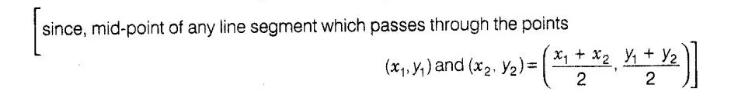# The point which lies on the perpendicular

Question:

The point which lies on the perpendicular bisector of the line segment joining the points A(-2, – 5) and B(2, 5) is

(a) (0,0)

(b) (0, 2)

(c) (2, 0)

(d)(-2,0)

Solution:

(a) We know that, the perpendicular bisector of the any line segment divides the^jjpe segment into two equal parts i.e., the perpendicular bisector of

the line segment always passes through the mid-point of the line segment.

Mid-point of the line segment joining the points A (-2, -5) and S(2, 5)

$=\left(\frac{-2+2}{2}, \frac{-5+5}{2}\right)=(0,0)$Hence, (0, 0) is the required point lies on the perpendicular bisector of the lines segment.